# Attractor

﻿
Attractor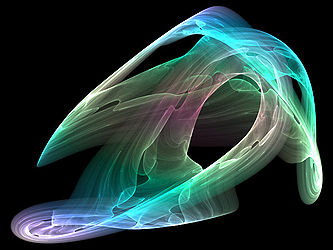Visual representation of a strange attractor

An attractor is a set towards which a dynamical system evolves over time. That is, points that get close enough to the attractor remain close even if slightly disturbed. Geometrically, an attractor can be a point, a curve, a manifold, or even a complicated set with a fractal structure known as a strange attractor. Describing the attractors of chaotic dynamical systems has been one of the achievements of chaos theory.

A trajectory of the dynamical system in the attractor does not have to satisfy any special constraints except for remaining on the attractor. The trajectory may be periodic or chaotic or of any other type. If this condition is met, but the flow in the neighbourhood is away from the set, the set is called a repeller (or repellor).

## Motivation

A dynamical system is generally described by one or more differential or difference equations. The equations of a given dynamic system specify its behavior over any given short period of time. To determine the system's behavior for a longer period, it is necessary to integrate the equations, either through analytical means or through iteration, often with the aid of computers.

Dynamical systems in the physical world tend to be dissipative: if it were not for some driving force, the motion would cease. (Dissipation may come from internal friction, thermodynamic losses, or loss of material, among many causes.) The dissipation and the driving force tend to combine to kill out initial transients and settle the system into its typical behavior. This one part of the phase space of the dynamical system corresponding to the typical behavior is the attracting section or attractee.

Invariant sets and limit sets are similar to the attractor concept. An invariant set is a set that evolves to itself under the dynamics. Attractors may contain invariant sets. A limit set is a set of points such that there exists some initial state that ends up arbitrarily close to the limit set (i.e. to each point of the set) as time goes to infinity. Attractors are limit sets, but not all limit sets are attractors: It is possible to have some points of a system converge to a limit set, but different points when perturbed slightly off the limit set may get knocked off and never return to the vicinity of the limit set.

For example, the damped pendulum has two invariant points: the point x0 of minimum height and the point x1 of maximum height. The point x0 is also a limit set, as trajectories converge to it; the point x1 is not a limit set. Because of the dissipation, the point x0 is also an attractor. If there were no dissipation, x0 would not be an attractor.

## Mathematical definition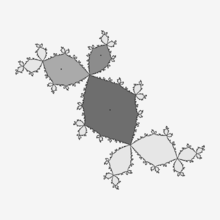Attracting period 3 cycle and its immediate basin of attraction

Let f(t, •) be a function which specifies the dynamics of the system. That is, if a is a point in the phase space, representing the initial state of the system, then f(0, a) = a and, for a positive value of t, f(t, a) is the result of the evolution of this state after t units of time. For example, if the system is a free particle in one dimension then the phase space is the plane R2 with coordinates (x,v), where x is the position of the particle and v is its velocity, and the evolution is given by$f(t,(x,v))=(x+tv,v).\$

An attractor is a subset A of the phase space characterized by the following three conditions:

• A is forward invariant under f: if a is an element of A then so is f(t,a), for all t > 0.
• There exists a neighborhood of A, called the basin of attraction for A and denoted B(A), which consists of all points b that "enter A in the limit t → ∞". More formally, B(A) is the set of all points b in the phase space with the following property:
For any open neighborhood N of A, there is a positive constant T such that f(t,b) ∈ N for all real t > T.
• There is no proper subset of A having the first two properties.

Since the basin of attraction contains an open set containing A, every point that is sufficiently close to A is attracted to A. The definition of an attractor uses a metric on the phase space, but the resulting notion usually depends only on the topology of the phase space. In the case of Rn, the Euclidean norm is typically used.

Many other definitions of attractor occur in the literature. For example, some authors require that an attractor have positive measure (preventing a point from being an attractor), others relax the requirement that B(A) be a neighborhood.[citation needed]

## Types of attractors

Attractors are parts of the phase space of the dynamical system. Until the 1960s, as evidenced by textbooks of that era, attractors were thought of as being geometrical subsets of the phase space: points, lines, surfaces, volumes. The (topologically) wild sets that had been observed were thought to be fragile anomalies. Stephen Smale was able to show that his horseshoe map was robust and that its attractor had the structure of a Cantor set.

Two simple attractors are the fixed point and the limit cycle. There can be many other geometrical sets that are attractors. When these sets (or the motions on them), are hard to describe, then the attractor is a strange attractor, as described in the section below.

### Fixed point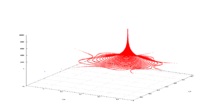Weakly attracting fixed point for complex quadratic polynomial

A fixed point is a point of a function that does not change under some transformation. If we regard the evolution of a dynamical system as a series of transformations, then there may or may not be a point which remains fixed under the whole series of transformation. In general there would not be such a point, but there may be one. The final state that a dynamical system evolves towards, such as the final states of a falling pebble, a damped pendulum, or the water in a glass corresponds to a fixed point of the evolution function, and will occur at the attractor, but the two concepts are not equivalent. A marble rolling around in a basin may have a fixed point in phase space even if it doesn't in physical space. Once it has lost momentum and settled into the bottom of the bowl it then has a fixed point in physical space, phase space, and is located at the attractor for that system.

### Limit cycle

See main article limit cycle

A limit cycle is a periodic orbit of the system that is isolated. Examples include the swings of a pendulum clock, the tuning circuit of a radio, and the heartbeat while resting. The ideal pendulum is not an example because its orbits are not isolated. In phase space of the ideal pendulum, near any point of a periodic orbit there is another point that belongs to a different periodic orbit.

### Limit tori

There may be more than one frequency in the periodic trajectory of the system through the state of a limit cycle. If two of these frequencies form an irrational fraction (i.e. they are incommensurate), the trajectory is no longer closed, and the limit cycle becomes a limit torus. We call this kind of attractor Nt-torus if there are Nt incommensurate frequencies. For example here is a 2-torus: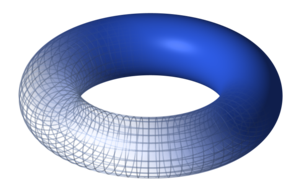A time series corresponding to this attractor is a quasiperiodic series: A discretely sampled sum of Nt periodic functions (not necessarily sine waves) with incommensurate frequencies. Such a time series does not have a strict periodicity, but its power spectrum still consists only of sharp lines.

### Strange attractor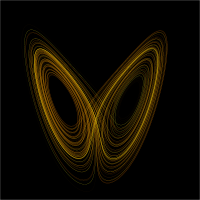A plot of Lorenz's strange attractor for values ρ=28, σ = 10, β = 8/3

An attractor is informally described as strange if it has non-integer dimension. This is often the case when the dynamics on it are chaotic, but there exists also strange attractors that are not chaotic. The term was coined by David Ruelle and Floris Takens to describe the attractor that resulted from a series of bifurcations of a system describing fluid flow. Strange attractors are often differentiable in a few directions, but some are like a Cantor dust, and therefore not differentiable.

Examples of strange attractors include the Hénon attractor, Rössler attractor, Lorenz attractor, Tamari attractor.

## Partial differential equations

Parabolic partial differential equations may have finite-dimensional attractors. The diffusive part of the equation damps higher frequencies and in some cases leads to a global attractor. The Ginzburg–Landau, the Kuramoto–Sivashinsky, and the two-dimensional, forced Navier–Stokes equations are all known to have global attractors of finite dimension.

For the three-dimensional, incompressible Navier–Stokes equation with periodic boundary conditions, if it has a global attractor, then this attractor will be of finite dimensions.

## Numerical localization (visualization) of attractors

From computation point of view, attractors can be naturally regarded as self-exciting attractors or hidden attractors. Self-exciting attractors can be localized numerically by standard computational procedure, in which after transient process a trajectory, started from a point of unstable manifold in a small neighborhood of unstable equilibrium, reaches an attractor and computes it (like classical attractors in Van der Pol, Beluosov–Zhabotinsky, Lorenz, and many others dynamical systems). While basin of attraction of hidden attractors does not contain neighborhoods of equilibria, and therefore hidden attractor cannot be localized by standard computational procedure.

Wikimedia Foundation. 2010.

### Look at other dictionaries:

• Attractor — At*tract or ([a^]t*tr[a^]kt [ e]r), n. One who, or that which, attracts. Sir T. Browne [1913 Webster] …   The Collaborative International Dictionary of English

• attractor — In non linear dynamic series, an attractor defines the equilibrium level of the system. Bloomberg Financial Dictionary See: point attractor, limit cycles, and strange attractor. Bloomberg Financial Dictionary …   Financial and business terms

• attractor — noun 1. an entertainer who attracts large audiences he was the biggest drawing card they had • Syn: ↑drawing card, ↑draw, ↑attraction, ↑attracter • Derivationally related forms: ↑attract ( …   Useful english dictionary

• attractor — 1) fish attractor (any structure placed in the water to create habitat for fishes) 2) a type of fly that is very effective but has little resemblance to a natural food item, usually very flashy and large …   Dictionary of ichthyology

• attractor — noun A set of points or states to which a dynamical system evolves after a long enough time. That is, points that get close enough to the attractor remain close even if slightly disturbed. See Also: basin of attraction, fixed point …   Wiktionary

• attractor — attract ► VERB 1) draw in by offering something interesting or advantageous. 2) cause (a specified reaction). 3) (often be attracted to) cause to have a liking for or interest in. 4) draw (something) closer by exerting a force. DERIVATIVES… …   English terms dictionary

• attractor — noun see attract …   New Collegiate Dictionary

• attractor — /euh trak teuhr/, n. 1. a person or thing that attracts. 2. Physics. a state or behavior toward which a dynamic system tends to evolve, represented as a point or orbit in the system s phase space. [1645 55] * * * …   Universalium

• attractor — at·trac·tor …   English syllables

• attractor epimeralis muscle — (ARTHROPODA: Crustacea) In Decapoda, a prominent muscle inserting along the line of the brachiocardiac groove …   Dictionary of invertebrate zoology